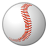# QlikView App Dev

Discussion Board for collaboration related to QlikView App Development.

Announcements
QlikWorld 2023, a live, in-person thrill ride. Save \$300 before February 6: REGISTER NOW!
cancel
Showing results for
Did you mean:Not applicable

## Aggregated average

Hello:

I have the following question. If I have the following table:

 city_id date red_cars total_cars 1 12/11/2015 10 50 1 12/12/2015 5 20 1 12/13/2015 0 10 2 12/11/2015 2 30

I would like to compute the average of red cars aggregated by the average by city.

For instance, {[(10 + 5 + 0)/(50 + 20 + 10)] + [(2/30)]} / 2

4 RepliesNot applicable
Author

Create a pivot table and add dimensions as your id,date and in the expression use avg(red_Cars)/total_cars

Thanks,

SVPartner - Specialist

You can use the following expression in a text object, for example:

=sum(aggr(sum(red_cars)/sum(total_cars),city_id))/count(DISTINCT city_id)

EduardoSpecialistSum(Aggr(Sum(red_cars),city_id)) = Sum all values for each city

Sum(Aggr(Sum(red_cars),city_id))/Sum(Aggr(Sum(total_cars),city_id)) = Divide Red_Cars by Total_CarsPartner - Specialist

If I understood the problem, the requirement was to obtain the average of the city of red_cars to total_cars ratio, and this is what my expression does

Look at the original expression

{[(10 + 5 + 0)/(50 + 20 + 10)] + [(2/30)]} / 2 results in 0.12708333

My expression, also resulted in 0.12708333

EduardoCommunity Browser